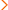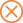Home > ed > ACT Scoring - How ACT Test Scoring Works

# ACT Scoring - How ACT Test Scoring Works

Before your child takes the ACT, it's a good idea for him or her to be familiar with how the ACT test scoring works. Here are the basics of what your child needs to know.

Scoring for the Multiple-Choice Sections (English, Math, Reading, and Science)

• Students earn 1 point for each correct answer and neither lose nor gain points for each omitted or incorrect answer.
• A student's raw score for a section is calculated by determining the number of questions answered correctly in that section. Example: If a student answered 60 questions correctly in the English section, his English raw score would be 60.
• A student's raw score for a section is converted to a scaled score, which ranges between a 1 and a 36, with 36 being the highest possible score. Students receive a scaled score for each of the four multiple-choice test sections (English, Math, Reading, and Science).
• A student's composite ACT score is the average of the student's scaled scores for the four multiple-choice test sections. Example: If a student scored a 24 English, 28 Math, 26 Reading, and 23 Science, his composite ACT score would be (24 + 28 + 26 + 23)/4 = 25.25, which is rounded down to a 25.

Scoring for the Writing Test

• Two readers read and score each student's Writing test essay based on four domains: Ideas and Analysis, Development and Support, Organization, and Language Use and Conventions.
• Each reader gives the essay a score ranging between a 1 and a 6 for each of the four domains, with 6 being the highest possible score. The two scores are added together to get a student's Writing subscore for each domain, which can range between a 2 and a 12 (12 being the highest possible score). If the two readers' scores differ by more than one point, a third reader will be called in to resolve the disagreement. The Writing score is calculated from the domain scores and ranges between 1 and 36.
• A student's English, Reading and Writing scores are scaled to create a student's combined English Language Arts (ELA) score. Choosing to take the Writing test does not impact your Composite score or subject area scores, but without the Writing test score, no ELA score will be reported.

Related Topics

• Find out why StudyPoint recommends viewing ACT scores before sending them to colleges. Visit our Sending ACT Scores page.
• For more specific information about the ACT format and question types, visit our ACT Test page.

LEARN MORE